Question

# A 3.00 kg ball is thrown vertically into the air with an initial velocity of 15.0 m/s

A 3.00 kg ball is thrown vertically into the air with an initial velocity of 15.0 m/s. The maximum height of the ball is

The ball will stop rising when the potential energy gain (M g H) equals the initial kinetic energy (M V^2/2).
Solve for H.

This is not college level physics

#### Earn Coins

Coins can be redeemed for fabulous gifts.

Similar Homework Help Questions
• ### A ball is thrown vertically upwards with an initial velocity of 27.0 m/s. Neglecting air resistance,...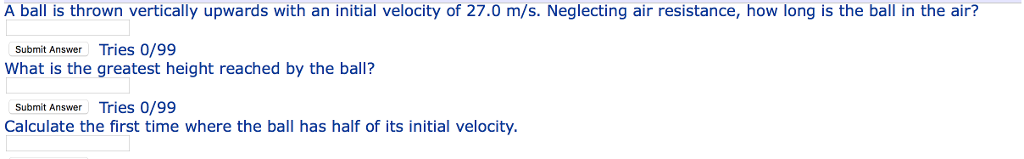A ball is thrown vertically upwards with an initial velocity of 27.0 m/s. Neglecting air resistance, how long is the ball in the air? Submit Answer Tries 0/99 What is the greatest height reached by the ball? Submit Answer Tries 0/99 Calculate the first time where the ball has half of its initial velocity

• ### A ball is thrown vertically upwards with an initial velocity of 18.80 m/s, from the ground....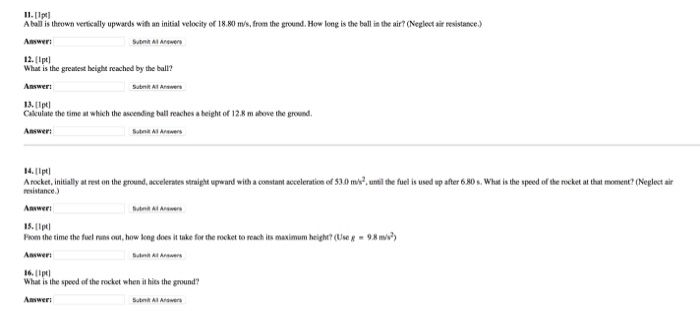A ball is thrown vertically upwards with an initial velocity of 18.80 m/s, from the ground. How long is the ball in the air? (Neglect air resistance.) What is the greatest height reached by the ball? Calculate the time at which the ascending ball reaches a height of 12.8 m above the ground. A rocket, initially at rest on the ground, accelerates straight upward with a constant acceleration of 53.0 m/s^2, until the fuel is used up after 6.80s. What...

• ### 9) A 2 kg object is thrown vertically upward with an initial velocity of 10 m/s....

9) A 2 kg object is thrown vertically upward with an initial velocity of 10 m/s. (a) What is its velocity at a point halfway to the maximum height it will attain? (a) What is the total time required for it to return to its initial position? (c) What is its acceleration at the maximum height? (d) What is its average speed during the complete trip? (neglect air resistance

• ### A ball is thrown straight upward in the air at a speed of 15.0 m/s. Ignore air resistance.

A ball is thrown straight upward in the air at a speed of 15.0 m/s. Ignore air resistance. a) What is the maximum height the ball will reach? b) What is the speed of the ball when it reaches 5.00 m?c) How long will the ball take to reach 5.00 m above its initial position on the way up? d) How long will the ball take to reach 5,00 m above its initial position on its way down?

• ### A basketball is thrown vertically upward with an initial velocity of 22.8 m/s. 'a) How long...

A basketball is thrown vertically upward with an initial velocity of 22.8 m/s. 'a) How long (s) does the ball take to reach its maximum height? 'b) How high (km) does the basketball go?

• ### A 0.09 kg ball of dough is thrown straight up into the air with an initial speed of 5.5 m/s

A 0.09 kg ball of dough is thrown straight up into the air with an initial speed of 5.5 m/s. (a) Find the momentum of the ball of dough at its maximum height. (b) Find the momentum of the ball of dough halfway to its maximum height on the way up.

• ### help A 0.20 kg ball is thrown straight up into the air with an initial speed...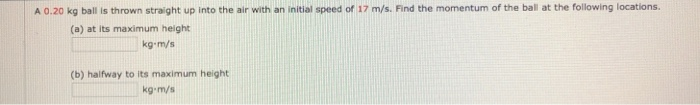help A 0.20 kg ball is thrown straight up into the air with an initial speed of 17 m/s. Find the momentum of the ball at the following locations. (a) at its maximum height kg-m/s (b) halfway to its maximum height kg-m/s

• ### A ball is thrown with in an initial velocity of 31.4 m/s straight up. What is...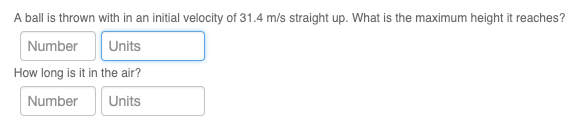A ball is thrown with in an initial velocity of 31.4 m/s straight up. What is the maximum height it reaches? Number Units How long is it in the air? NumberUnits

• ### The height(h) of an object that has been dropped or thrown in the air is given by: h(t)=-4.9t^2+vt+h t=time in seconds(s) v=initial velocity in meters per second (m/s) h=initial height in meters(m) A ball is thrown vertically upwardd from the top of the

The height(h) of an object that has been dropped or thrown in the air is given by: h(t)=-4.9t^2+vt+ht=time in seconds(s)v=initial velocity in meters per second (m/s)h=initial height in meters(m)A ball is thrown vertically upwardd from the top of the Leaning Tower of Pisa (height=53m) with an initial velocity of 30m/s. Find the time(s) at which:a) the ball's height equals the hight of the towerb) the ball's height is greater than the height of the towerc) the ball's height is less...

• ### Ba Example 2-16 Ball thrown upward A woman throws a ball upward into the air with...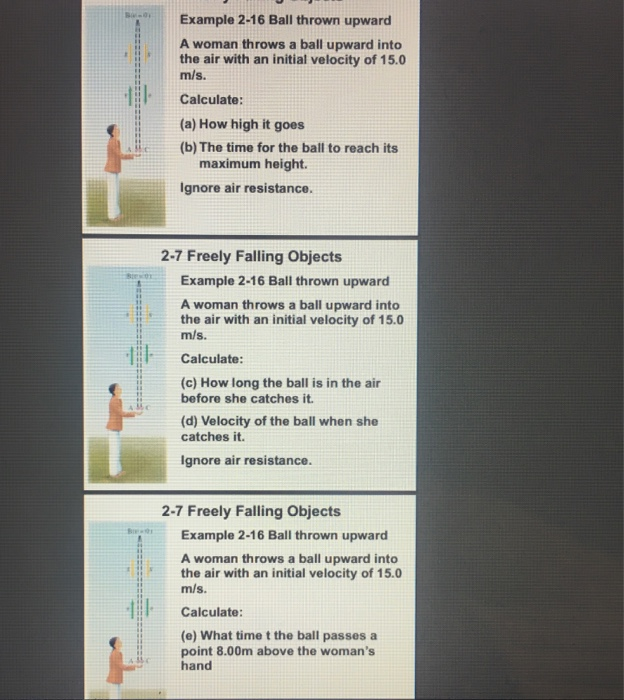Ba Example 2-16 Ball thrown upward A woman throws a ball upward into the air with an initial velocity of 15.0 m/s. Calculate: (a) How high it goes (b) The time for the ball to reach its maximum height Ignore air resistance. 2-7 Freely Falling Objects Example 2-16 Ball thrown upward A woman throws a ball upward into the air with an initial velocity of 15.0 m/s. Calculate: (c) How long the ball is in the air before she catches...